« STRUCTURAL FRAME LOADING Seismic loads » Gravity Loads The loads applied to a structure are divided into permanent (dead loads) and imposed (live loads). The former include the self weight of reinforced-concrete structural members, of walls and coatings-coverings. The latter include loading caused by people, furniture, vehicles etc. Dead loads The density and the related unit weight of the materials used in construction are: Reinforced concrete ρ=2.50 t/m3 (ε=25.0 kN/m3) Light- weight concrete for ground leveling ρ=0.80 t/m3 (ε=8.0 kN/m3) Sand mortar ρ=2.00 t/m3 (ε=20.0 kN/m3) Marble ρ=2.70 t/m3 (ε=27.0 kN/m3)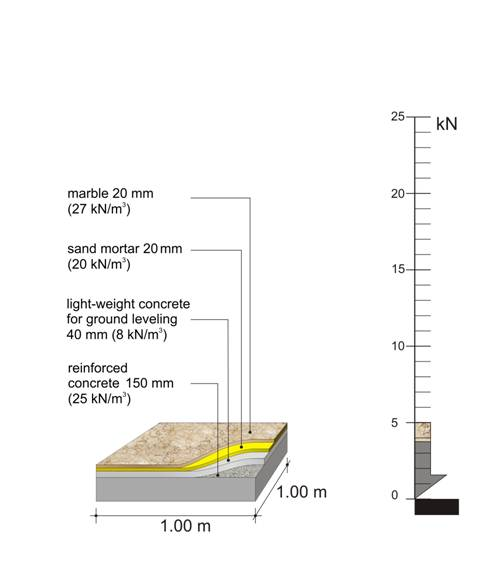The dead mass of one m2 of the above slab is, g = 0.15*2.50 + 0.04*0.8 + 0.02*2.0 + 0.02*2.7 = 0.5 t, i.e. the self mass of one square meter of a usual slab is 0.5 t (weight 5.0 kN) The dead mass of one m2 of the above slab is, g = 0.15*2.50 + 0.04*0.8 + 0.02*2.0 + 0.02*2.7 = 0.5 t, i.e. the self mass of one square meter of a usual slab is 0.5 t (weight 5.0 kN) Water ρ = 1.00 t/ m3 (ε = 10.0 kN/m3)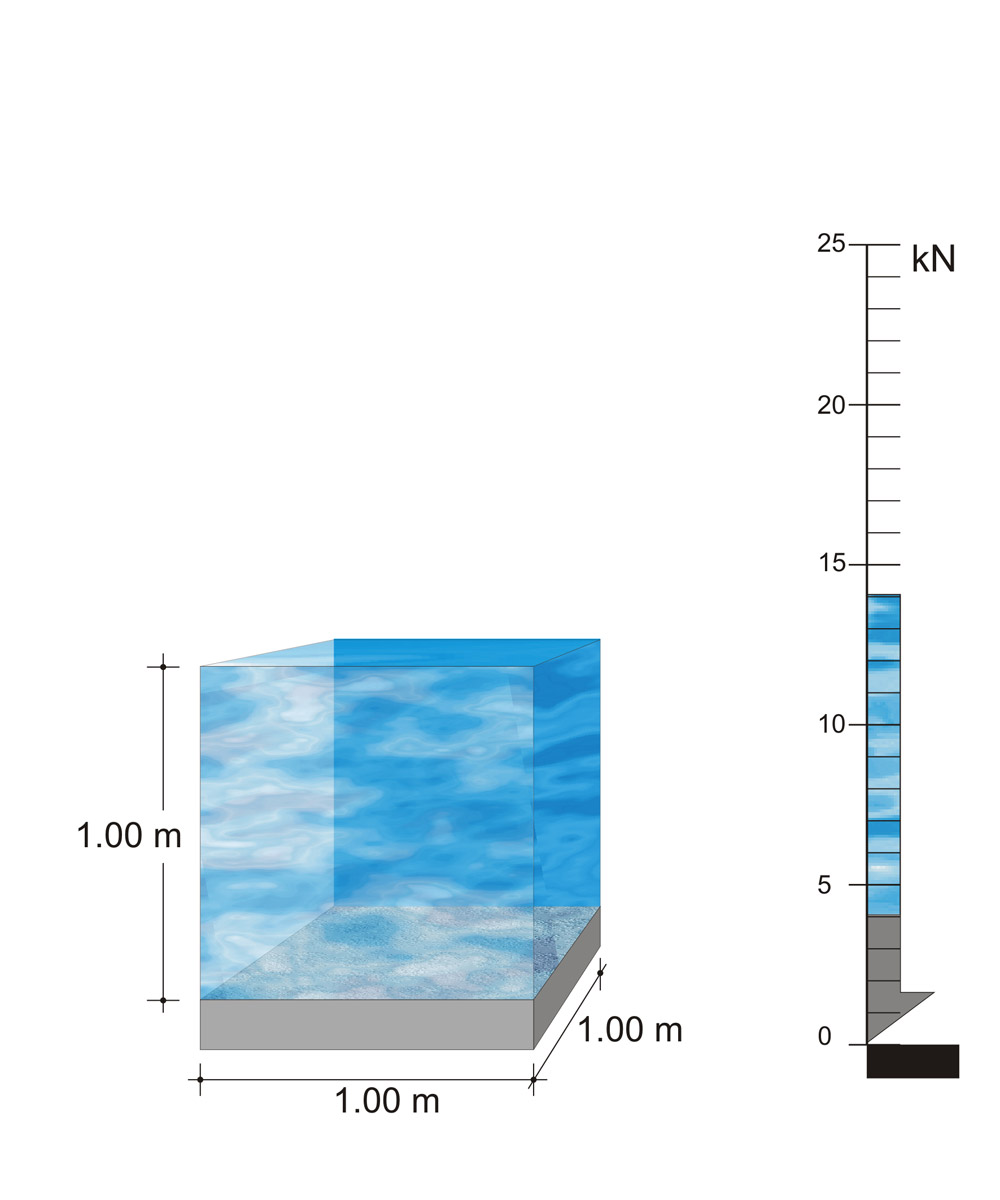The dead mass of one m2 of a pool slab, when the pool is filled with just 1.0 m of water is, 1.4 t (weight 14.0 kN) The dead mass of one m2 of a pool slab, when the pool is filled with just 1.0 m of water is, 1.4 t (weight 14.0 kN) Garden soil ρ = 2.50 t/m3 (ε = 25.0 kN/m3)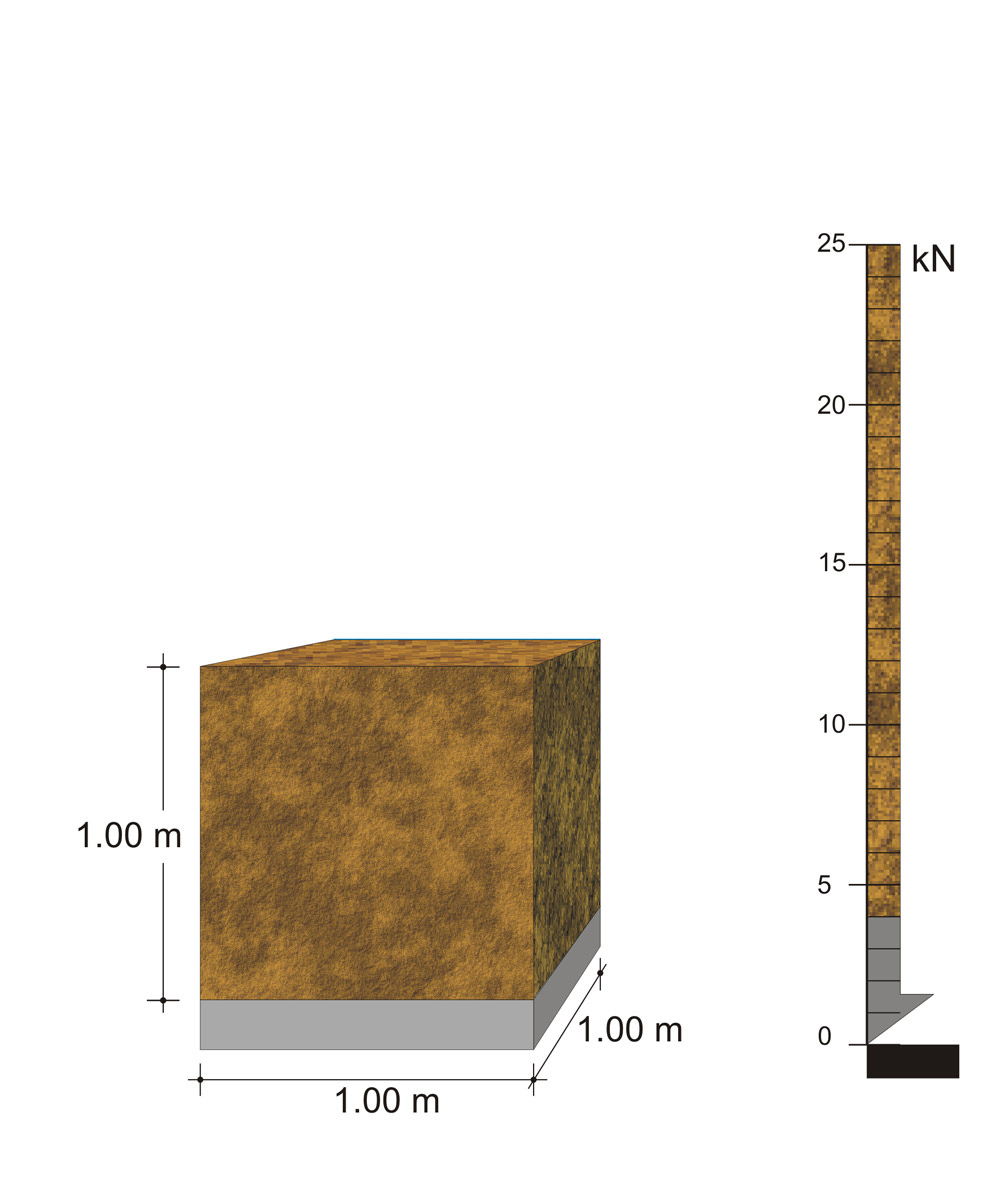The dead mass of one m2 of a slab, with 1.0 m of soil on top is 2.5 t (weight 25.0 kN The dead mass of one m2 of a slab, with 1.0 m of soil on top is 2.5 t (weight 25.0 kN Masonry stretcher bond ρ = 0.21 t/m² (ε = 2.1 kN/m²) Masonry Flemish bond ρ = 0.36 t/m² (ε = 3.6 kN/m²)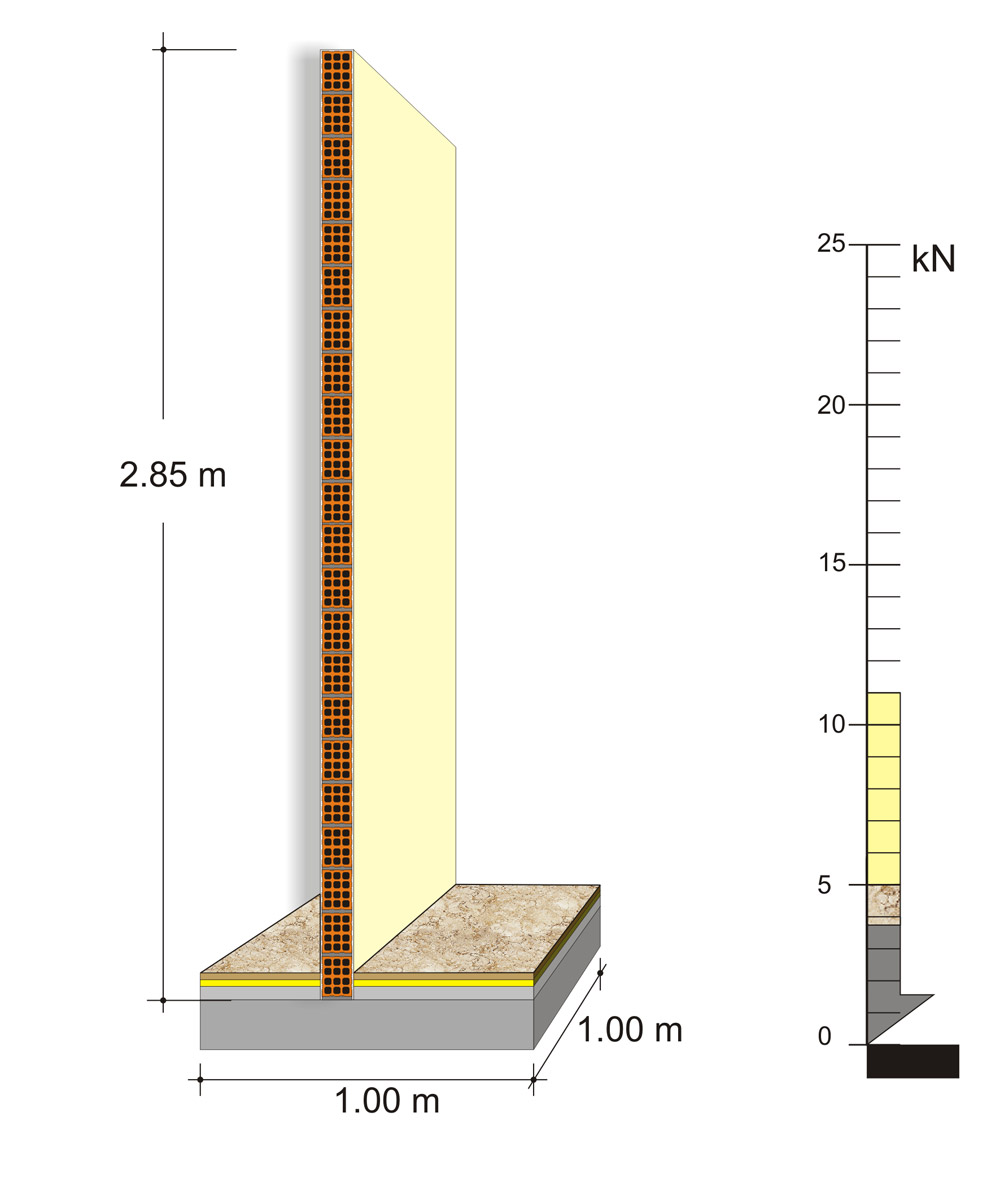A wall of 1.00 m length, 2.85 m height and 100 mm thickness has a mass equal to 0.6 t. (weight 6.0 kN) A wall of 1.00 m length, 2.85 m height and 100 mm thickness has a mass equal to 0.6 t. (weight 6.0 kN) Live loads Human loading: - Normal human loading ρ = 0.20 t/m² (ε = 2.0 kN/m²)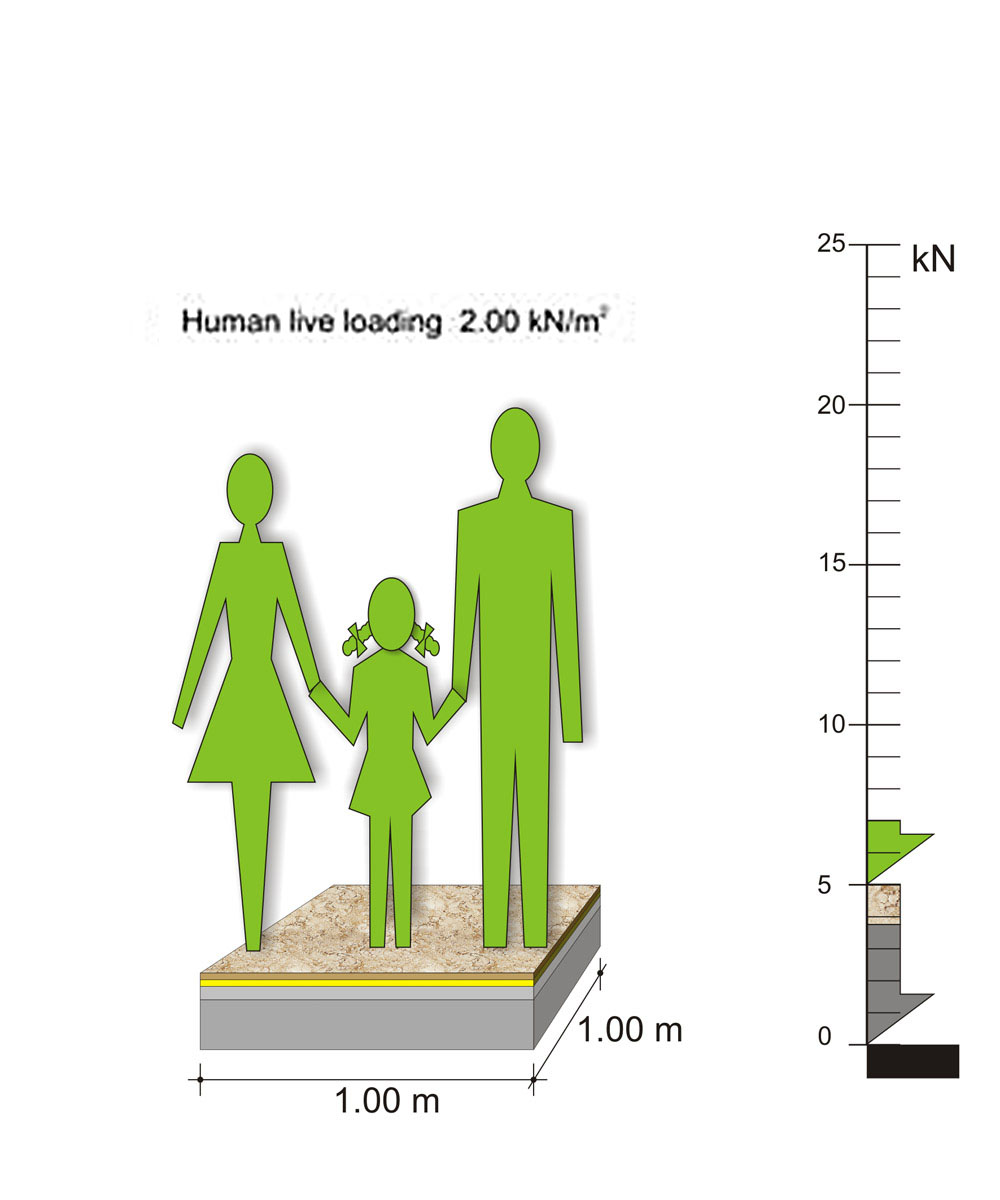The live mass of one m2 residential building is 0.2 t (weight 2.0 kN) The live mass of one m2 residential building is 0.2 t (weight 2.0 kN) - Humans concentration ρ = 0.50 t/m² (ε = 5.0 kN/m²)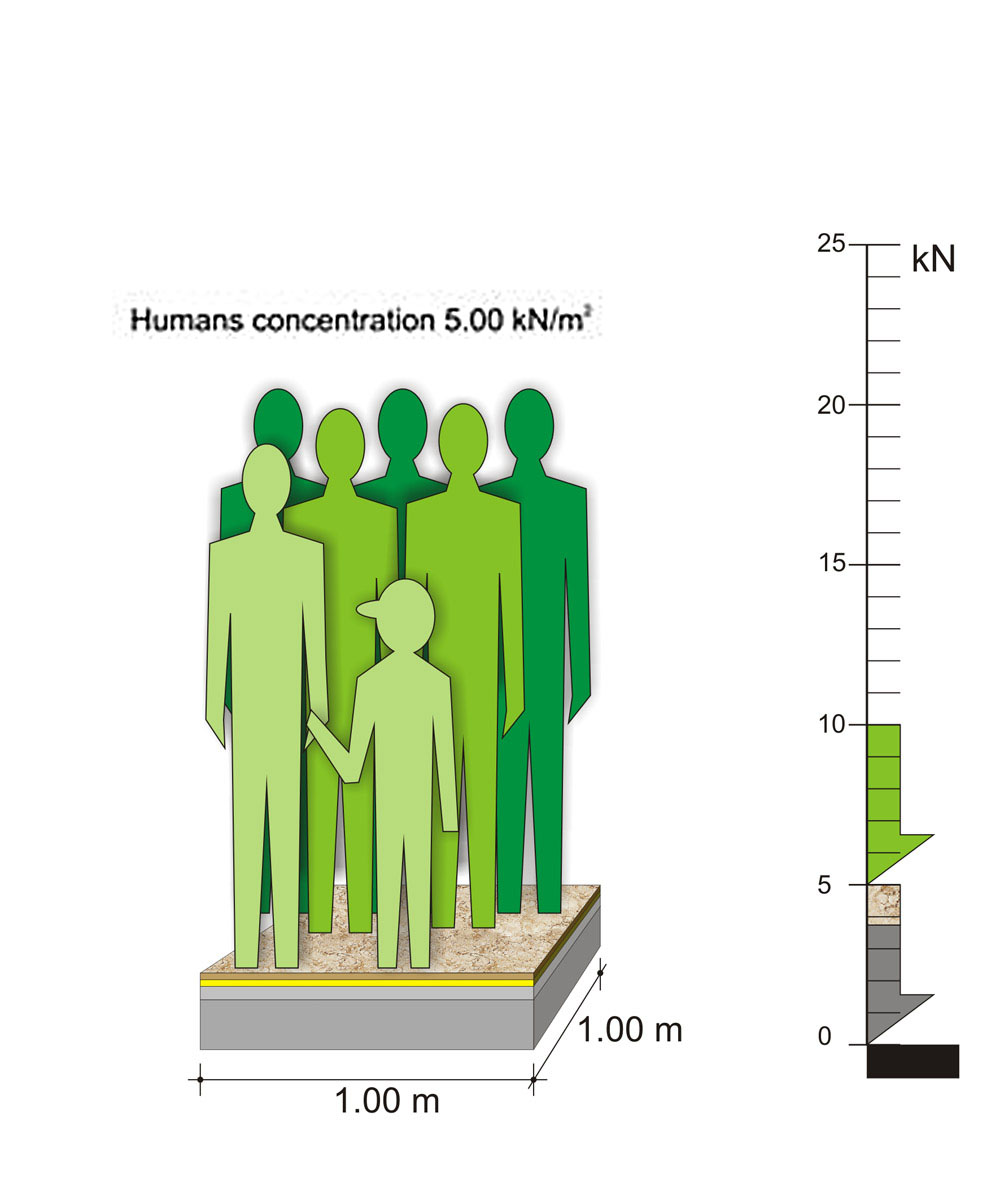The live mass of one m² commercial area is 0.5 t (weight 5.0 kN) The live mass of one m² commercial area is 0.5 t (weight 5.0 kN) Vehicle loading ρ = 0.25 t/m² (ε = 2.5 kN/m²)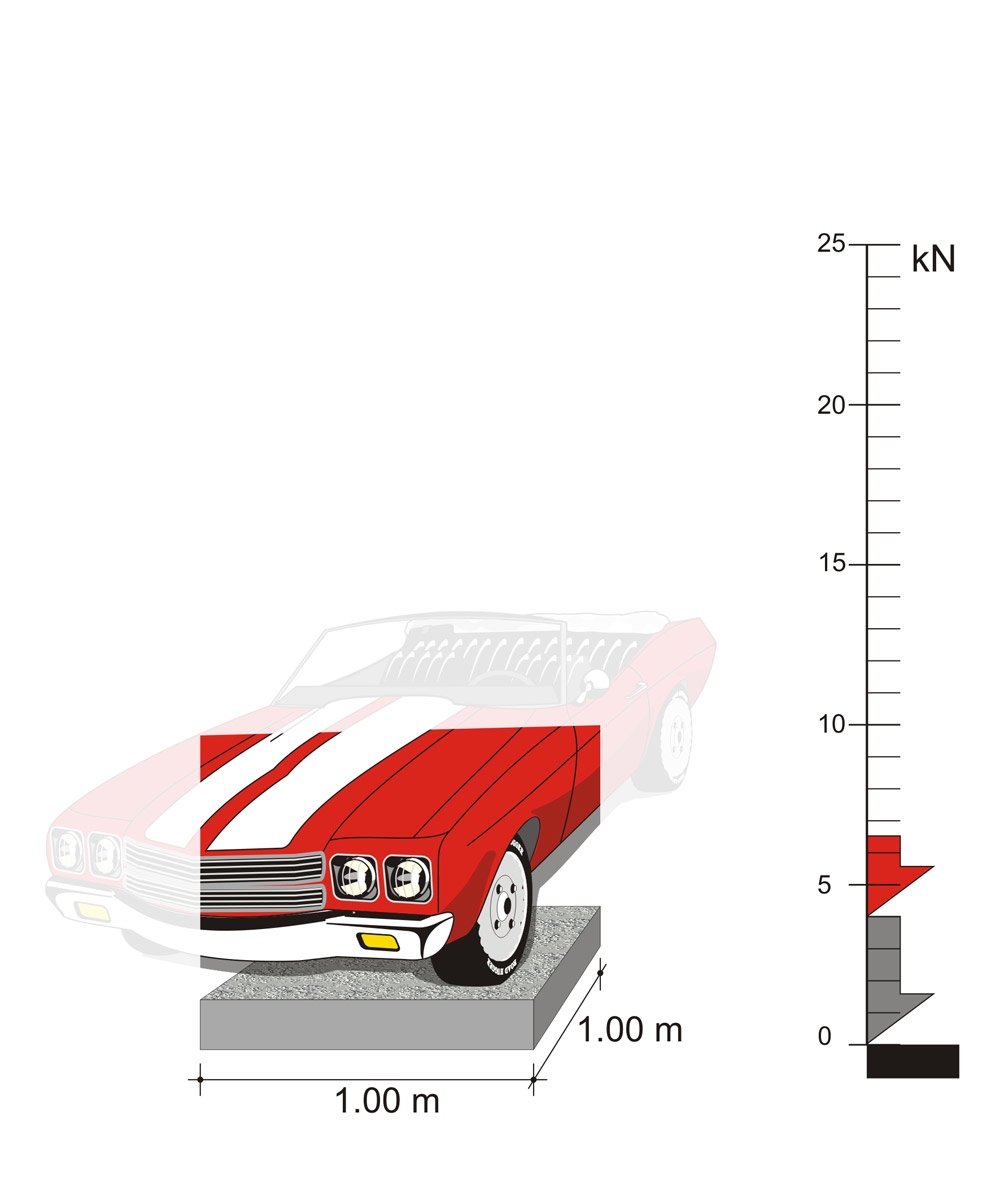The live distributed load of 1 m² of a parking space is 0.25 t (weight 2.5 kN) The live distributed load of 1 m² of a parking space is 0.25 t (weight 2.5 kN) As a rule, snow loading is lower than the live load generated by the use of people and its value ranges between 0.60 and 1.50 kN/m². Note : In order to calculate the amount of the total loading applied to a residential structure we assume 1 m² of slab i.e. 1 m x 1 m surface. In every 1 m² of floor surface, the permanent (dead) and the induced (live) masses, are in the order of 0.50 t (weight 5.0 KN) and 0.20 t (weight 2.0 KN) respectively. However when we consider the loads applied by beams, columns, walls and plasters, the total amount of the dead loads i.e. the structure’s self weights, is greater than 10 kN/m², as opposed to the live loads that remain equal to 2 kN/m². Moreover in a specific time in the structure’s life span, the possible extensive permanent loads may be around 100% in contrast to the possible extensive induced loads that may be around 30%. From all the above we realize how greater are the dead loads in comparison to the live ones and this is one of the most important problems of structures, they “use” to many dead loads in order to bear a few live loads.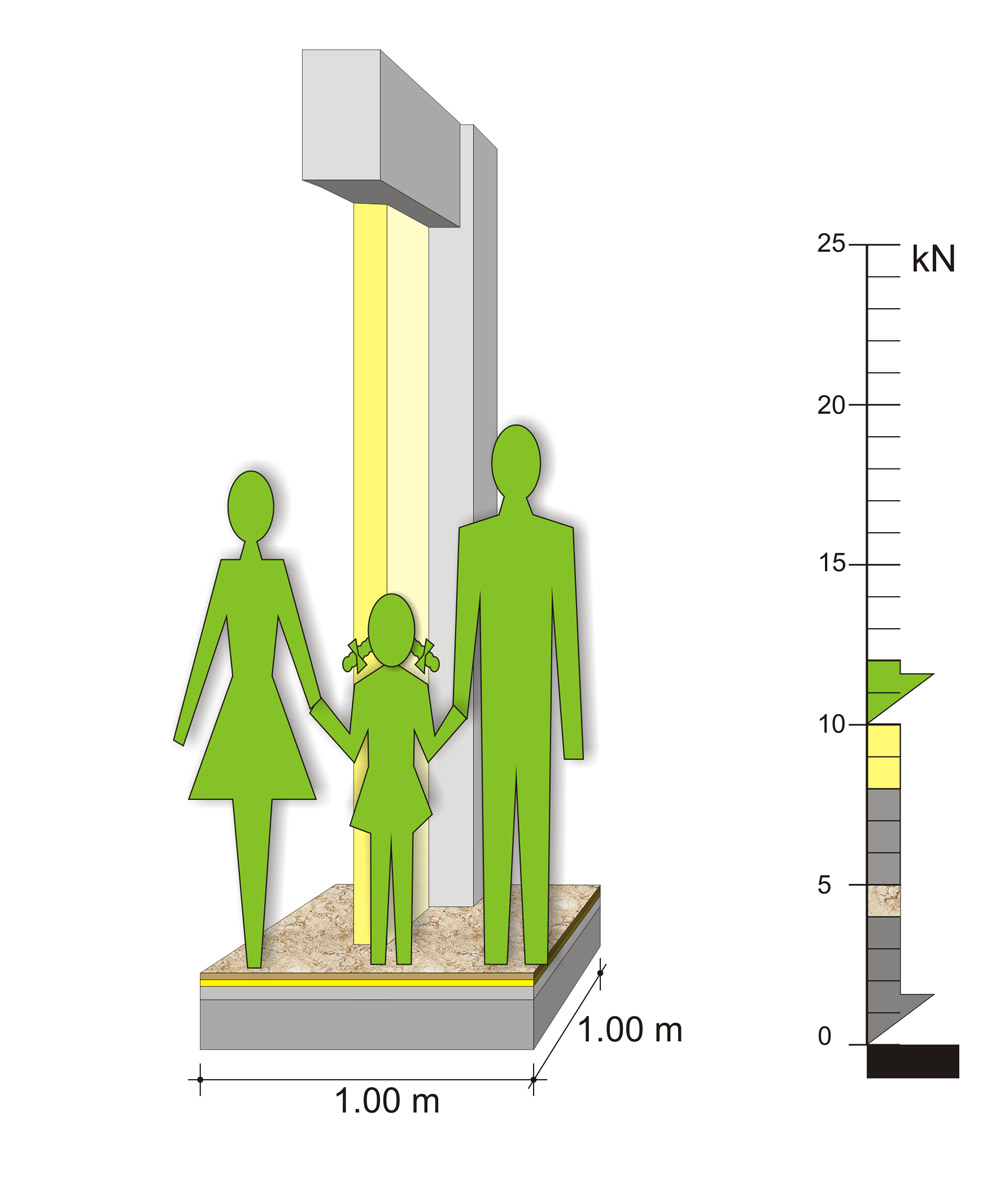In a residential building, the maximum live loads are about 20% of the dead loads. In a residential building, the maximum live loads are about 20% of the dead loads.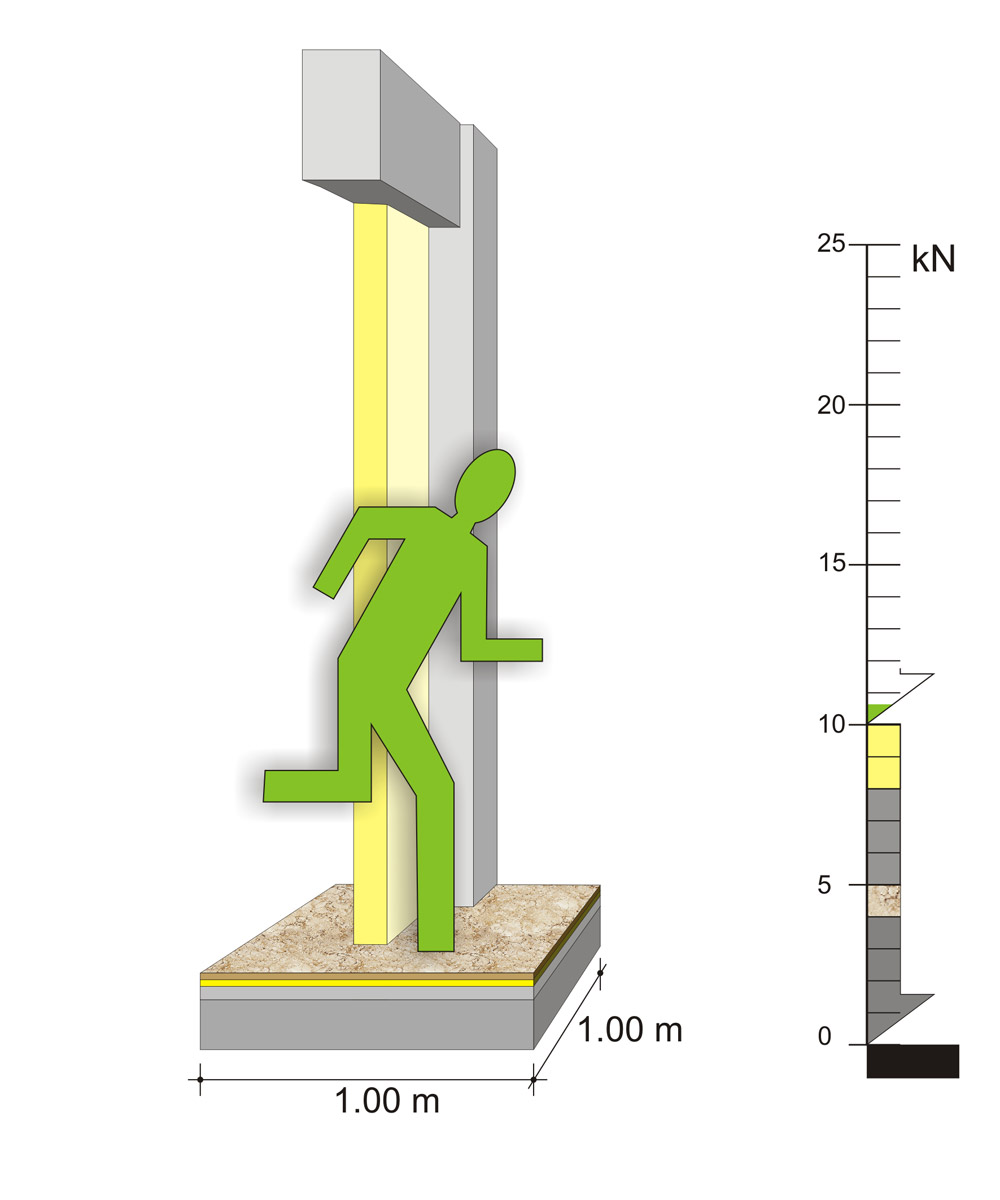In random cases like earthquakes, the extensive live loads may reach 6% of the dead loads. In random cases like earthquakes, the extensive live loads may reach 6% of the dead loads. « STRUCTURAL FRAME LOADING Seismic loads »